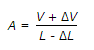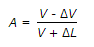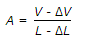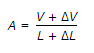# Civil Engineering - Soil Mechanics and Foundation Engineering

### Exercise :: Soil Mechanics and Foundation Engineering - Section 6

6.

The area of cross-section A at failure or during any stage of Triaxial Compression Test and its initial length (L) and volume (V), are related by the equation

 A.B.C.D.Explanation:

No answer description available for this question. Let us discuss.

7.

Pick up the correct statement from the following:

 A. In hydrometer method, weight Wd per ml of suspension is found directly B. In pipette analysis, weight Wd per ml of suspension is found indirectly C. In pipette analysis, weight Wd per ml of suspension is found directly D. None of these.

Explanation:

No answer description available for this question. Let us discuss.

8.

Pick up the correct statement from the following:

 A. Coefficient of compressibility is the decrease in void ratio per unit increase of pressure B. The percent settlement at any time is called degree of consolidation C. Time factor is a dimensionless quantity D. The initial curve on either side of the point of unloading and reloading is called 'virgin curve' E. All the above.

Explanation:

No answer description available for this question. Let us discuss.

9.

'Loess' is silty clay formed by the action of

 A. water B. glacier C. wind D. gravitational force.

Explanation:

No answer description available for this question. Let us discuss.

10.

The maximum possible value nf dry density is referred to as

 A. dry density B. zero air voids C. saturation dry density D. all the above.